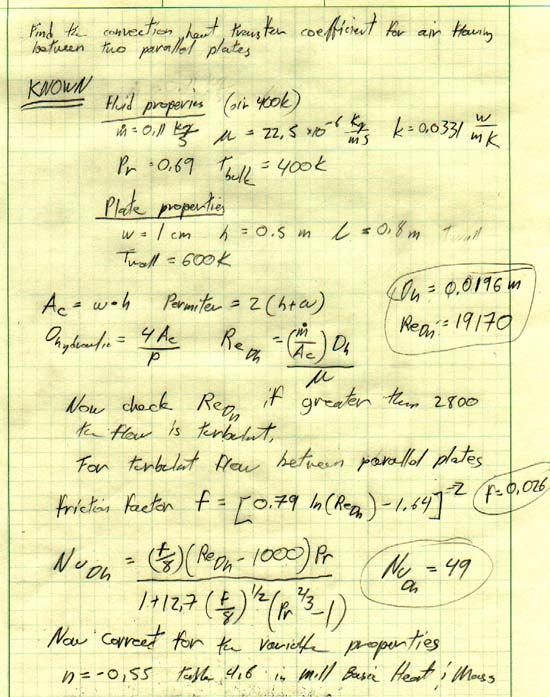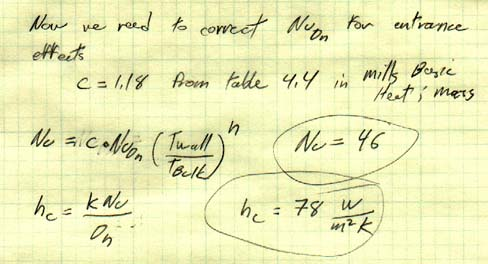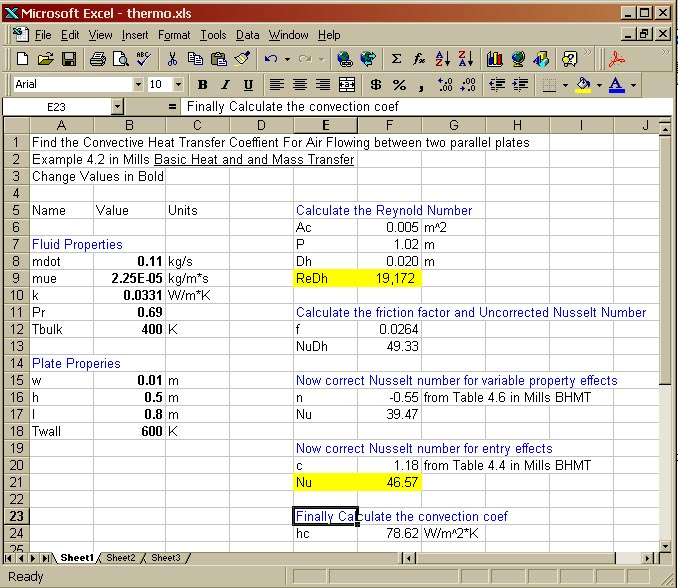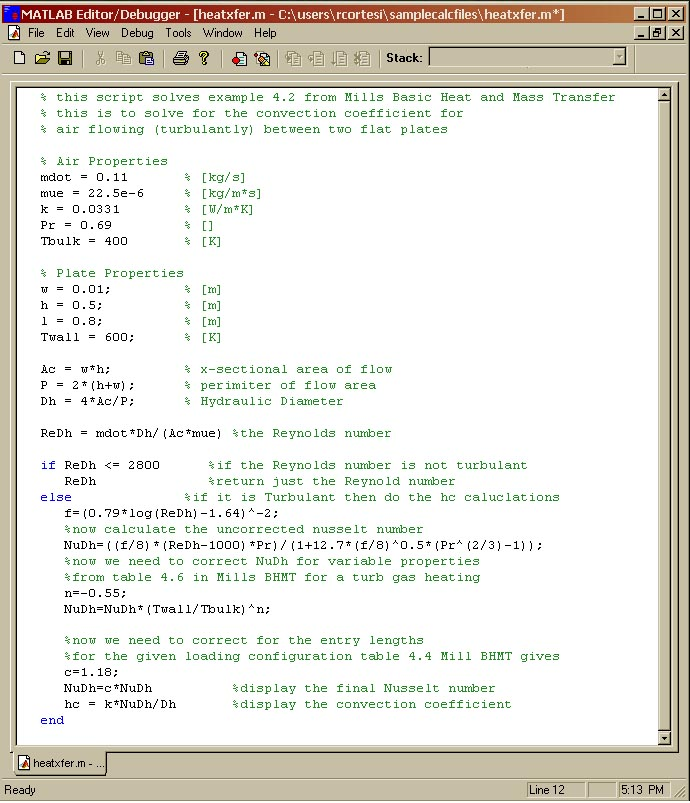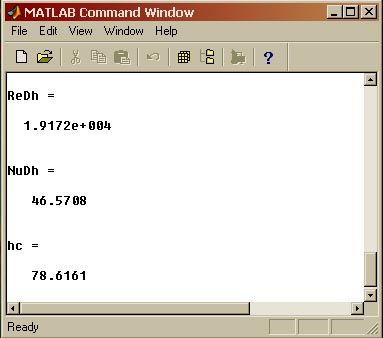# Design Cookbook: Using Software Packages for Engineering Calculations

It is very common for students to have trouble when completing their engineering calculations.  Professor Sachs at MIT has written some excellent guidelines for doing calculations.  READ THEM AND TAKE THEM TO HEART.  This document is intended to show you some examples of how to clearly present your work in the various software packages.  It also points out the pro's, con's, and pitfalls of each.

### Why use a software package when doing calculations?

2. Suffer through the pain of working out the math for a particular problem once.  Save the file and you can use it as a resource later.
3. If you do make a mistake, you can correct the single mistake and the rest of the numbers afterward will correct themselves.
4. You can compare several designs quickly (why waist your time plugging and chugging through a much of math.
5. And don't forget that matrices and linear algebra are your friends when solving systems of linear independent equations.

There are three basic packages available at MIT for doing basic engineering calculations.  They are:

It is the easiest to read, complicated equations can be typed in very quickly, and it keeps track of units for you.  These three features make it very easy to write, catch errors, and grade.   Very easy to enter and solve linear systems.

### Microsoft Excel:

This is good for displaying multiple designs side by side.  How ever if you use it in this manner you can not take advantage of the ability to give the cells meaningful names.  Grading or reviewing a design done in excel is difficult because you can't see the equations that were used.  So, you have to have additional paperwork to show the equations used for each cellt.  Very tedious to enter and solve linear systems.

### MATLAB:

Good for doing big numeric analysis. Code can be difficult to read, understand, and grade if it is not commented well, and variables are not given meaningful names.  Very nice plotting features.  Good at solving linear systems, but entering the matrices can be a pain.

## Examples Problems:

Below are some example problems showing a good method of presenting your work in the various software packages.  What you hand in should be very clear and easy to follow. To do well on your calculations keep the following in mind.  Have a clear idea of what you know and what you are looking for.  State these clearly at the top of your calculation.

### Example Problem 1:

This is Example 4.2 from A. F. Mill's Basic Heat and Mass Transfer (BHMT).

We are interested in finding the coefficient of convection for an air stream traveling between two plates.  The plates have a temperature of 600 K and the average bulk temperature of the air is 400 K.  The air is flowing at 0.11 kg/s, and the channel between the plates is 1 cm wide and 0.5 meters high.  The channel is 0.8 meters long.  The entrance has a 90 degree edge.

MathCAD is far and away my favorite package (of the three above) for heat transfer problems.  This is due to the amount of unit checking that is needed and the complexity of the equations that are typically involved.  If I wanted to test a different plate size and the values were given in inches, I could just enter the new values for w, h, and l (in inches), and keep the fluid parameters in metric units (much more convenient to look up in metric) and it would calculate hc correctly.

NOTE: If you are using units in MathCAD make sure you don't give variables the same name as a unit that you are using. For example if you are using the unit of meters (m) then do not also denote the mass of an object with the variable m.  This will really screw things up.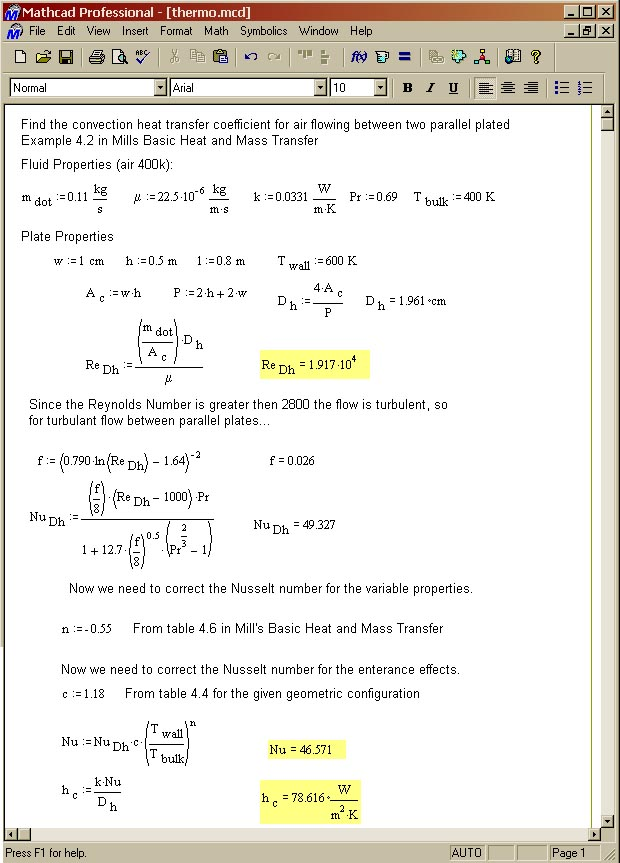#### The Solution in Excel:

The following is an example of a well laid out Excel spread sheet.   It produces the exact same solution as the MathCAD file above, however...

• When I'm grading it I can't see the equations that are used to generate the values in each cell.  So an accompanying equation sheet needs to be scanned in and posted as well.
• Be very careful with units in Excel.  I always enter everything in meters, and N/m^2 etc. not mm, GPa etc.  If you make entries in mm and GPA is is really easy to forget to include the appropriate factors of 10 in your equations and then you are off by several thousand or million (a bad thing).
• Also, in these spreadsheets, I always make bold any cells that I can enter values in.  It is just too easy in big spread sheets to forget which cells are calculating values and which aren't.
• It is also really easy to forget parentheses when entering your equations.
• Another pet peeve of mine with spread sheets is when people leave about 14 significant digits in their calculations.  A cell that reads 0.23879852 meters really only needs to read 0.2388 at the most, and when every cell in the sheet has 14 significant digits it becomes difficult to read the sheet.

First I do the calculation out by hand.  This ensures that I understand the problem, and that I am not simply the plugging things blindly into cells.  It also give an easy way to check the spread sheet.  Given the same inputs I should get the same result as the hand calculation.  Doing the calculations by hand first, is much less important in MathCAD because units are tracked automatically and it is easy to read the equations.